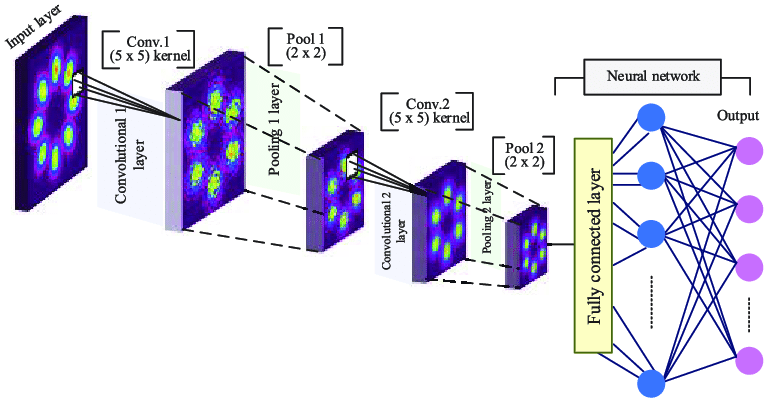# For a beginner how to start with CNN using MNIST Dataset

Original article can be found here (source): Deep Learning on Medium# For a beginner how to start with CNN using MNIST Dataset

Introduction:

In deep learning, a convolutional neural network (CNN, or ConvNet) is a class of deep neural networks, most commonly applied to analyzing visual imagery. They are also known as shift invariant or space invariant artificial neural networks (SIANN), based on their shared-weights architecture and translation invariance characteristics.

They have applications in image and video recognition, recommender systems, image classification, medical image analysis, natural language processing, and financial time series.

Convolutional networks were inspired by biological processes in that the connectivity pattern between neurons resembles the organization of the animal visual cortex.

Individual cortical neurons respond to stimuli only in a restricted region of the visual field known as the receptive field. The receptive fields of different neurons partially overlap such that they cover the entire visual field.

Architecture

A convolutional neural network consists of an input and an output layer, as well as multiple hidden layers. The hidden layers of a CNN typically consist of a series of convolutional layers that convolve with multiplication or other dot product.

The activation function is commonly a ReLU layer and is subsequently followed by additional convolutions such as pooling layers, fully connected layers and normalization layers, referred to as hidden layers because their inputs and outputs are masked by the activation function and final convolution.

Convolution:

Convolution is a generalized way of multiplication it is similar to dot product performed on matrices.

In convolution, the image is filtered with a small kernel or filter which reduce the size of the picture without losing the relationship between pixels. Let’s say the convolution image is (3*3) is multiplied with kernel size=(2*2).

Then the convolution of (3*3) image matrix multiplies with (2*2) filter matrix which is called Feature Map.

-Pooling

Pooling layers subsample their input. Pooling size is to reduce the amount of the parameters by selecting the maximum, average, or sum values inside these pixels. The most common way to do pooling it to apply a max operation to the result of each filter. Pooling reduces the output dimensionality.

Pooling gives invariance to translation, rotation, scaling.

Translation invariance: Wherever the face is should be able to locate it.

Rotational invariance: Whether the face is straight or titled should be able to locate it.

Scale invariance: Whether the face is small or big should be able to locate it.

Zero Padding: The nice feature of zero padding is that it will allow us to control the spatial size of the output volumes ( we will use it to exactly preserve the spatial size of the input volume so the input and output width and height are the same).

Valid Padding: Drop the part of the image where the filter did not fit. This is called valid padding which keeps only valid part of the image.

-Strides

Stride is the number of pixels shifts over the input matrix. When the stride is 1 then we move the filters to 1 pixel at a time. When the stride is 2 then we move the filters to 2 pixels at a time and so on. The below figure shows convolution would work with a stride of 2.

Padding and strides are used to leverage the strides of multiplication.

Activation unit:

tanh, sigmoid, ReLU is used for activation as Relu has better performance it is used mostly

Implementation of CNN on MNIST dataset

Now let’s consider MNIST dataset. Each image is a 28 by 28-pixel squares with greyscale. There are 10 digits (0 to 9) or 10 classes to predict.

Import the required libraries: